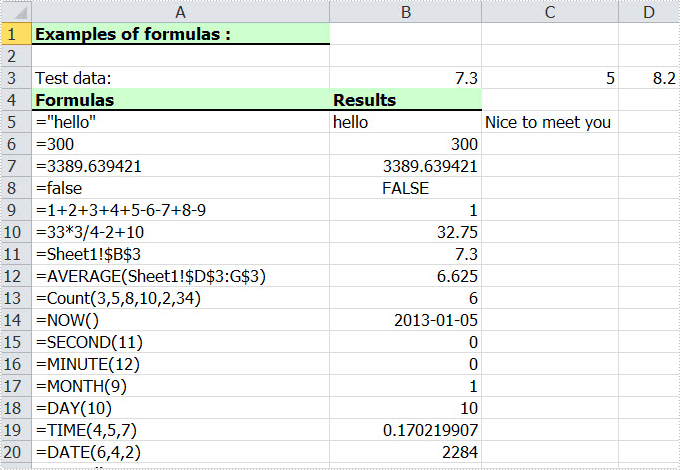# Add Excel Formulas in C#, VB.NET

In an Excel Worksheet, we may import a great deal of data. Sometimes, we need to calculate the data to get other numbers we need. It is a time consuming job when we deal with large spreadsheets and more complex data. Formula is the main tool to calculate data. There are various formulas included in Excel. Below I will show you how to add formula in C#, VB.NET by Spire.XLS.

Spire.XLS .NET is a Professional and stable .NET Excel component which enables developers/programmers to operate Excel files with their ASP.NET web sites and Windows Forms applications. It supports calculate complex Excel Formulas. Spire.XLS presents you an easiest way to add formula for Excel.

Please check the effective screenshot first.Now we will give you a demo with many kinds of formulas written in the worksheet. The formula can be string, bool value, calculation, sheet area reference, time and so on. Here, we first reference a variable "currentRow" to control rows of all kinds of formulas. Second, reference "currentFormula". Assign value for Worksheet.Range [cellRange]. Text to write a formula, and then via currentFormula to assign value for Worksheet.Range [cellRange]. Formula to get the formula results.

[C#]
```using Spire.Xls;

namespace Formula
{
class Program
{
static void Main(string[] args)
{
//Create a new workbook
Workbook workbook = new Workbook();

//Initialize worksheet
Worksheet sheet = workbook.Worksheets;

//initialize currentRow
int currentRow = 3;
string currentFormula = string.Empty;

//test data
sheet.Range[currentRow, 2].NumberValue = 7.3;
sheet.Range[currentRow, 3].NumberValue = 5; ;
sheet.Range[currentRow, 4].NumberValue = 8.2;
sheet.Range[currentRow, 5].NumberValue = 4;
sheet.Range[currentRow, 6].NumberValue = 3;
sheet.Range[currentRow, 7].NumberValue = 11.3;

//string.
currentFormula = "=\"hello\"";
sheet.Range[++currentRow, 1].Text = "=\"hello\"";
sheet.Range[currentRow, 2].Formula = currentFormula;

//bool.
currentFormula = "=false";
sheet.Range[++currentRow, 1].Text = currentFormula;
sheet.Range[currentRow, 2].Formula = currentFormula;

//calculation
currentFormula = "=33*3/4-2+10";
sheet.Range[++currentRow, 1].Text = currentFormula;
sheet.Range[currentRow, 2].Formula = currentFormula;

//sheet area reference
currentFormula = "=AVERAGE(Sheet1!\$D\$3:G\$3)";
sheet.Range[++currentRow, 1].Text = currentFormula;
sheet.Range[currentRow, 2].Formula = currentFormula;

//time
currentFormula = "=NOW()";
sheet.Range[++currentRow, 1].Text = currentFormula;
sheet.Range[currentRow, 2].Formula = currentFormula;
sheet.Range[currentRow, 2].Style.NumberFormat = "yyyy-MM-DD";

//Save the file
workbook.SaveToFile("Sample.xls",ExcelVersion.Version97to2003);

//Launch the file
System.Diagnostics.Process.Start("Sample.xls");
}
}
}
```
[VB.NET]
```Imports Spire.Xls

Module Module1

Sub Main()
'Create a new workbook
Dim workbook As New Workbook()

'Initialize worksheet
Dim sheet As Worksheet = workbook.Worksheets(0)

'initialize currentRow
Dim currentRow As Integer = 3
Dim currentFormula As String = String.Empty

'test data
sheet.Range(currentRow, 2).NumberValue = 7.3
sheet.Range(currentRow, 3).NumberValue = 5

sheet.Range(currentRow, 4).NumberValue = 8.2
sheet.Range(currentRow, 5).NumberValue = 4
sheet.Range(currentRow, 6).NumberValue = 3
sheet.Range(currentRow, 7).NumberValue = 11.3

'string.
currentFormula = "=""hello"""
sheet.Range(currentRow, 2).Formula = currentFormula

'bool.
currentFormula = "=false"
sheet.Range(currentRow, 2).Formula = currentFormula

'calculation
currentFormula = "=33*3/4-2+10"
sheet.Range(currentRow, 2).Formula = currentFormula

'sheet area reference
currentFormula = "=AVERAGE(Sheet1!\$D\$3:G\$3)"
sheet.Range(currentRow, 2).Formula = currentFormula

'time
currentFormula = "=NOW()"
sheet.Range(currentRow, 2).Formula = currentFormula
sheet.Range(currentRow, 2).Style.NumberFormat = "yyyy-MM-DD"

'Save doc file.
workbook.SaveToFile("Sample.xls",ExcelVersion.Version97to2003)

'Launching the MS Word file.
System.Diagnostics.Process.Start("Sample.xls")

End Sub
End Module
```

Spire.XLS supports many methods to add a formula for Excel including different parts. As a professional .NET Excel component, it owns the ability of inserting content into Excel document, formatting cells and converting Excel documents to popular office file formats. Spire.XLS for WPF supports Excel 97-2003, Excel 2007 and Excel 2010.